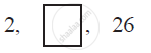Share

# In the following APs, find the missing terms in the boxes - CBSE Class 10 - Mathematics

#### Question

In the following APs, find the missing terms in the boxes :#### Solution

For this A.P.,

a = 2

a3 = 26

We know that, an = a + (n − 1) d

a3 = 2 + (3 − 1) d

26 = 2 + 2d

24 = 2d

d = 12

a2 = 2 + (2 − 1) 12

= 14

Therefore, 14 is the missing term

Is there an error in this question or solution?

#### APPEARS IN

NCERT Solution for Mathematics Textbook for Class 10 (2019 to Current)
Chapter 5: Arithmetic Progressions
Ex. 5.20 | Q: 3.1 | Page no. 106
Solution In the following APs, find the missing terms in the boxes Concept: nth Term of an AP.
S